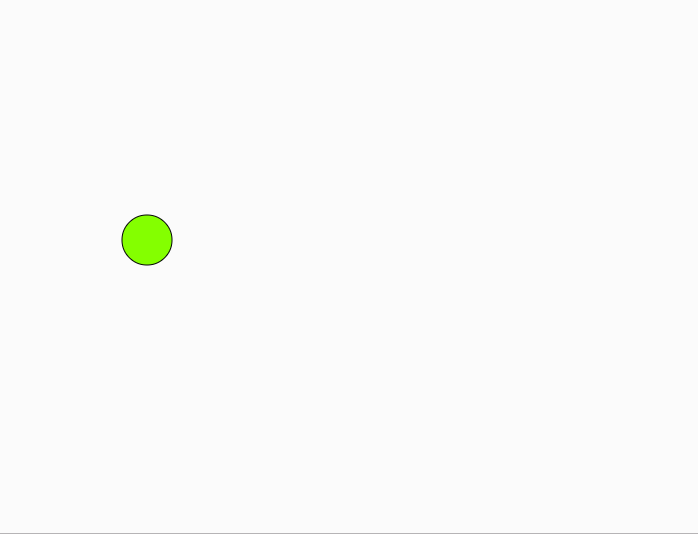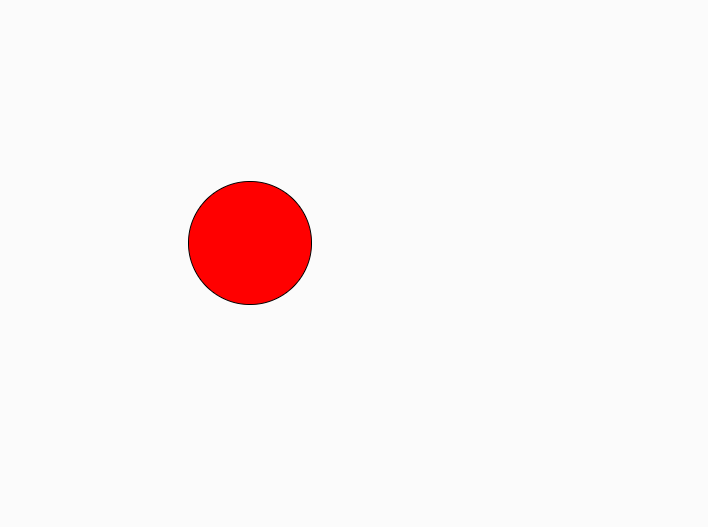# p5.js | Keyboard | keyIsDown()

• Last Updated : 16 Apr, 2019

The keyIsDown() function in p5.js checks the key’s current status that key is down, i.e. pressed. It can be used if you have a movable object, and you want several keys to be able to affect its behavior simultaneously, such as moving a sprite diagonally.

Syntax:

Hey geek! The constant emerging technologies in the world of web development always keeps the excitement for this subject through the roof. But before you tackle the big projects, we suggest you start by learning the basics. Kickstart your web development journey by learning JS concepts with our JavaScript Course. Now at it's lowest price ever!

```keyIsDown()
```

Below program illustrates the keyIsDown() function in p5.js:
Example-1:

 `let x = 100;``let y = 100;`` ` `function` `setup() {``   ` `    ``// create canvas of size 600*600``    ``createCanvas(600, 600);``}`` ` `function` `draw() {``   ` `    ``// fill color``    ``fill(x, y, x - y);`` ` `    ``if` `(keyIsDown(LEFT_ARROW)) {``        ``x -= 5;``    ``}`` ` `    ``if` `(keyIsDown(RIGHT_ARROW)) {``        ``x += 5;``    ``}`` ` `    ``if` `(keyIsDown(UP_ARROW)) {``        ``y -= 5;``    ``}`` ` `    ``if` `(keyIsDown(DOWN_ARROW)) {``        ``y += 5;``    ``}`` ` `    ``clear();``    ``ellipse(x, y, 50, 50);``}`

Output:Example-2:

 `let diameter = 30;`` ` `function` `setup() {``   ` `    ``// Create canvas of size 600*600``    ``createCanvas(600, 600);``}`` ` `function` `draw() {``   ` `    ``// 107 and 187 are keyCodes for "+"``    ``if` `(keyIsDown(107) || keyIsDown(187)) {``        ``diameter += 1;``    ``}`` ` `    ``// 109 and 189 are keyCodes for "-"``    ``if` `(keyIsDown(109) || keyIsDown(189)) {``        ``diameter -= 1;``    ``}`` ` `    ``clear();``    ``fill(255, 0, 0);``    ``ellipse(width / 2, height / 2, diameter, diameter);``}`

Output:Reference: https://p5js.org/reference/#/p5/keyIsDown

My Personal Notes arrow_drop_up